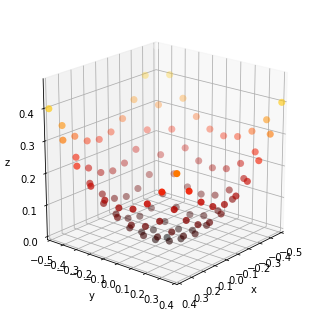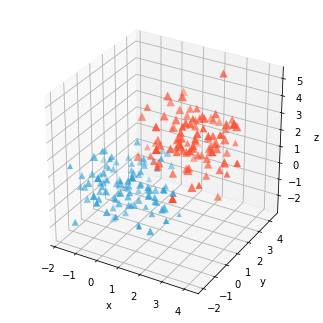# Scatter3d¶

Title
Scatter3D Element
Dependencies
Matplotlib
Backends
Matplotlib
Plotly
In :
import numpy as np
import holoviews as hv
from holoviews import dim, opts

hv.extension('matplotlib')Scatter3D represents three-dimensional coordinates which may be colormapped or scaled in size according to a value. They are therefore very similar to Points and Scatter types but have one additional coordinate dimension. Like other 3D elements the camera angle can be controlled using azimuth, elevation and distance plot options:

In :
y,x = np.mgrid[-5:5, -5:5] * 0.1
heights = np.sin(x**2+y**2)
hv.Scatter3D((x.flat, y.flat, heights.flat)).opts(
opts.Scatter3D(azimuth=40, elevation=20, color='z', s=50, cmap='fire'))

Out:Just like all regular 2D elements, Scatter3D types can be overlaid and will follow the default color cycle:

In :
(hv.Scatter3D(np.random.randn(100,4), vdims='Size') * hv.Scatter3D(np.random.randn(100,4)+2, vdims='Size')).opts(
opts.Scatter3D(s=(5+dim('Size'))*10, marker='^'))

Out:For full documentation and the available style and plot options, use hv.help(hv.Scatter3D).